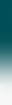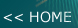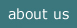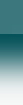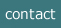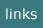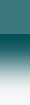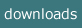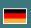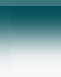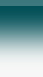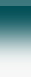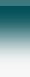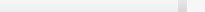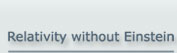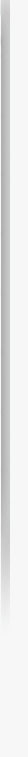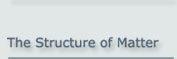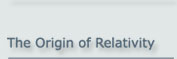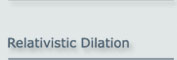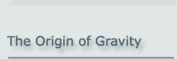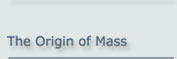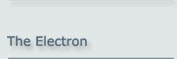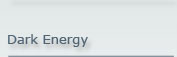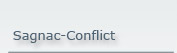The Sagnac effect disproves Einstein

The Sagnac effect, particularly its technical application as a laser gyroscope, is in clear conflict with Einstein's interpretation of Special Relativity.

The compatibility between Special Relativity and the Sagnac experiment has been repeatedly investigated and confirmed. In these cases, however, the Sagnac experiment was investigated for an observer who is at rest with respect to the experiment. In this case, there is no problem. However, if the Sagnac process is investigated for an observer who is rotating with the Sagnac set-up, then there is a conflict in his frame of reference. This conflict is usually refuted using the argument that Special Relativity only applies to inertial systems in linear motion, not to rotating systems. It can, however, be shown that a continuous transition is possible from a rotating Sagnac set-up to a system in linear motion.

Imagine the following set-up: We envision a small section of the optical fiber in the Sagnac loop. Parallel to this section, we envisage a short, straight section of a light path that is not curved. This section moves tangentially in the direction of the section of the rotating fiber loop under investigation. The speed of the surface of the fiber is designated v, the straight section moves at the same tangential speed v. See figure 1.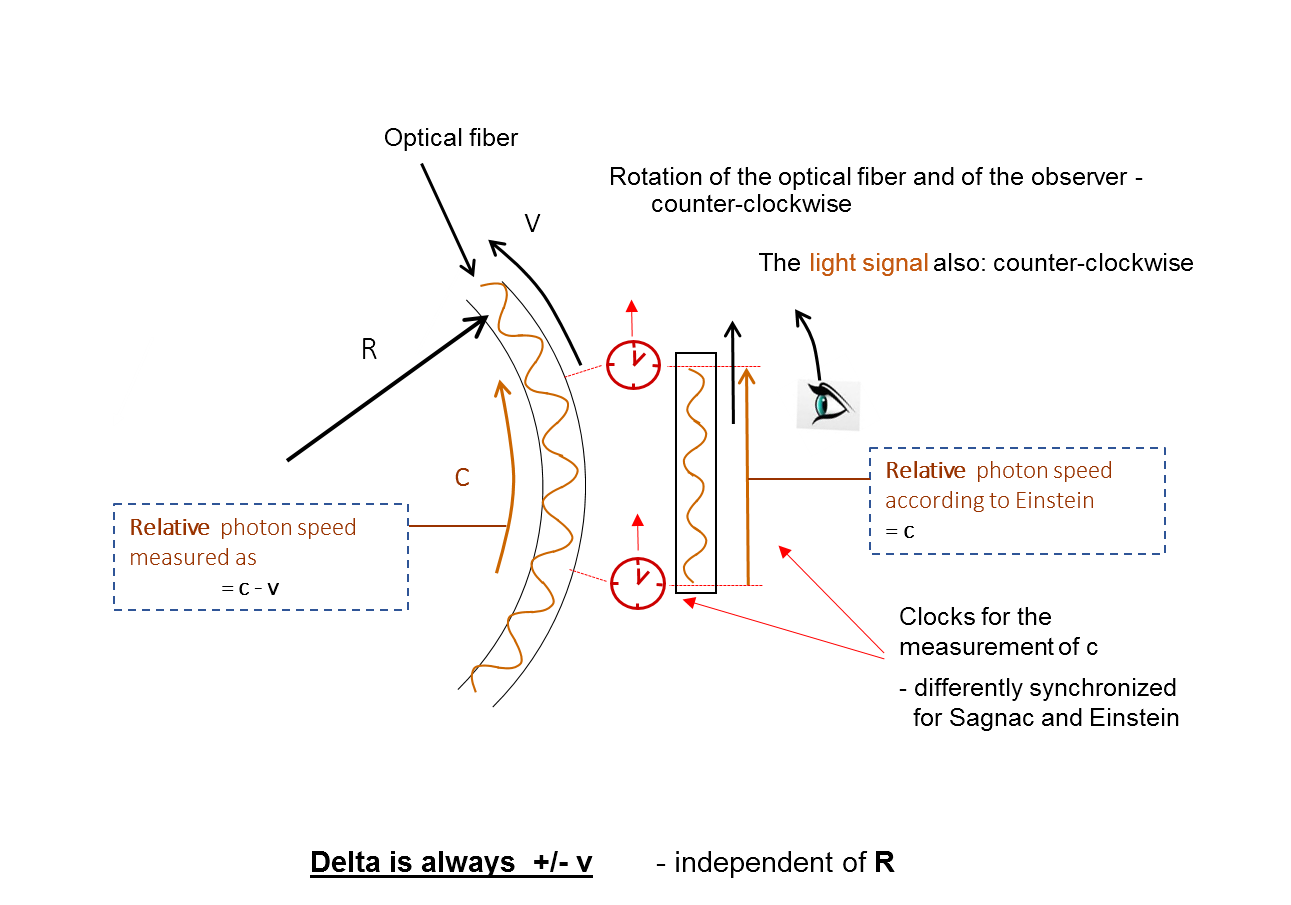Figure 1: Section of the rotating Sagnac gyroscope

For the sake of simplicity, we will assume that the speed of light in the fiber is the nominal c. The surface of the fiber along a curved path is close to the straight section and so temporarily parallel to the straight section.

What about the measured speed of light? Inside the fiber, the speed of light as seen by the moving observer is c+v or c-v, depending on the direction of the signal in the fiber. This is the result of measuring the speed of light along the full circuit. By symmetry, this speed has to be the same for any section of the circuit.

In the straight section (which is also moving), the speed of light is always c, according to Einstein. So we see a discrepancy here between the Sagnac fiber and Einstein's prediction. This is normally not accepted as a discrepancy because the Sagnac system is rotating and Special Relativity does not cover rotating systems. However, we can allow the two cases to converge.

Let's assume that the Sagnac system is made larger and larger, i.e. its radius increases. Its rotation is adjusted such that the speed at the surface remains v. The difference between Sagnac and Einstein therefore remains constant, at = +/- v.

If the radius of the Sagnac system is now extended to approximate infinity, then this algebraic difference remains v whereas the physical difference between the Sagnac section and the straight section vanishes. - In the limit then, we have two results for the same situation. Now we may ask, which result is correct? The answer is that the Sagnac result is correct because it can be directly measured, and it is the reason why this set-up can be used in navigation. So Einstein's solution cannot be correct. But why is it wrong?

This can be explained if we look into Einstein's deduction in his paper of 1905. Einstein defines clock synchronization, and he does it by assuming that the time that a light signal needs to travel from one clock to another is equal to the time needed in the opposite direction. This assumption leads to the commonly accepted result that the speed of light is constant in any inertial system. Einstein admits that this is in fact an assumption, however "free from contradictions". The thought experiment based on Sagnac described above shows that it does in fact lead to a logical conflict. So, the conclusion is that Einstein is wrong in this respect, and this in turn means that, in the end, the speed of light is NOT constant in all inertial systems.

Einstein's conclusion that the speed of light is constant in all inertial frames is a standard case of circular reasoning.

Here is Einstein's original text - translated into English:

"If at the point A of space there is a clock, an observer at A can determine the time values of events in the immediate proximity of A by finding the positions of the hands which are simultaneous with these events. If there is at the point B of space another clock in all respects resembling the one at A, it is possible for an observer at B to determine the time values of events in the immediate neighborhood of B. But it is not possible without further assumption to compare, in respect of time, an event at A with an event at B. We have so far defined only an "A time" and a "B time." We have not defined a common "time" for A and B, for the latter cannot be defined at all unless we establish by definition that the "time" required by light to travel from A to B equals the "time" it requires to travel from B to A. Let a ray of light start at the "A time" tA from A towards B, let it at the "B time" tB be reflected at B in the direction of A, and arrive again at A at the "A time" t'A.

In accordance with definition, the two clocks synchronize if tB - tA = t'A - tB

We assume that this definition of synchronism is free from contradictions, … "

(Emphasis in the text by the author.)

-------------------------------------------------------------------------------------------------------------------------------------------

(Subtitle: The Sagnac effect falsifies Special Relativity)

This site as a pdf-file